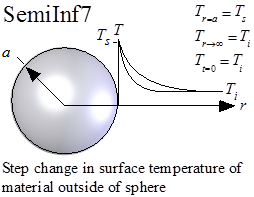Contents - Index

Step-change in Temperature for Semi-Infinite Body Outside of SphereFunction SemiInf7(T_i, T_s, alpha, a, r, time) returns the temperature within a semi-infinite that is located outside of a spherical surface and is subjected to a step change in the surface temperature.  Solution is from Carslaw and Jaeger as reported in the Handbook of Heat Transfer.

The calling protocol is:

T = SemiInf7(T_i, T_s, alpha, a, r, time)

Inputs:
T_i = initial temperature of the material [C] or [K]
T_s = surface temperature at r = a [C] or [K]
alpha = thermal diffusivity [m^2/s]
a = radius of sphere [m]
time = time relative to beginning of surface disturbance [s]

This function can be used with English units set in EES.  In this case, T_i and T_s are in [F] or [R], alpha is in [ft^2/hr], a is in [ft], r is in [ft], and time is in [s].

Outputs:
T is the temperature in [C] or [K] (or [F] or [R] in English units)

Rohsenow, W.M., J.P. Hartnett, and Y.I. Cho, Handbook of Heat Transfer, 3rd Edition, McGraw Hill, (1998).

Example:
\$UnitSystem SI Mass J C Pa
T_i = 100 [C]
T_s=200 [C]
alpha=1e-4 [m^2/s]
a = 0.01 [m]
r = 0.02 [m]
time=20 [s]
T = semiinf7(T_i,T_s, alpha, a, r, time)

{Solution:
T=143.7 [C]}

Transient Conduction Index